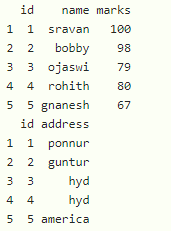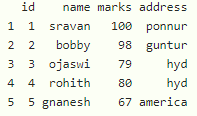Open in App
Not now

# Keep Original Row Order when Merging DataFrame in R

• Last Updated : 14 Sep, 2021

In this article, we are going to merge the dataframe by keeping the original row in the R programming language.

Dataframe in use:## Method 1: Using inner_join()

We can get the ordered merged dataframe by using an inner join. This function is available in dplyr package thus first we need to load the package. inner_join() is used to perform the inner join. It takes two dataframes as input and join the two dataframes based on the common column in both the dataframes.

Syntax:

inner_join(dataframe1,dataframe2)

where

• dataframe1 is the first dataframe
• dataframe2 is the second dataframe

Example: R program to merge two dataframe

## R

 `# load the package``library``(``"dplyr"``)`` ` `# create dataframe1 with id,name and marks``data1 = ``data.frame``(id=``c``(1, 2, 3, 4, 5),``                   ``name=``c``(``"sravan"``, ``"bobby"``, ``"ojaswi"``,``                          ``"rohith"``, ``"gnanesh"``),``                   ``marks=``c``(100, 98, 79, 80, 67))`` ` `# create dataframe2 with id and address``data2 = ``data.frame``(id=``c``(1, 2, 3, 4, 5),``                   ``address=``c``(``"ponnur"``, ``"guntur"``, ``"hyd"``,``                             ``"hyd"``, ``"america"``))`` ` `# merge the dataframes``inner_join``(data1, data2)`

Output:## Method 2: Using merge() function

merge() function is used to merge the dataframes.

Syntax:

merge(dataframe1,dataframe2)

where

• dataframe1 is the first dataframe
• dataframe2 is the second dataframe

Example: R program to merge the dataframes using merge() function

## R

 `# create dataframe1 with id,name and marks``data1 = ``data.frame``(id=``c``(1, 2, 3, 4, 5),``                   ``name=``c``(``"sravan"``, ``"bobby"``, ``"ojaswi"``, ``                          ``"rohith"``, ``"gnanesh"``),``                   ``marks=``c``(100, 98, 79, 80, 67))`` ` `# create dataframe2 with id and address``data2 = ``data.frame``(id=``c``(1, 2, 3, 4, 5),``                   ``address=``c``(``"ponnur"``, ``"guntur"``, ``"hyd"``, ``                             ``"hyd"``, ``"america"``))`` ` `# merge the dataframes using merge function``merge``(data1, data2)`

Output:My Personal Notes arrow_drop_up